In this example, the RANS and LES turbulence models were used to calculate the combustion of premixed gas. The steady-state calculation results of RANS and the statistical average results of LES were compared and analyzed. Among them, Figure 6 is a comparison of the results of the average velocity field. From Figure 6(a), it can be seen that in the LES statistical average result, there will be an obvious recirculation zone near the axis of the combustion chamber, and the steady calculation result of RANS This phenomenon is not obvious. As can be seen from the partial results of the velocity average field in Fig. 6(b), in the statistical average result of LES, after the airflow passes through the cyclone, there will be a significant backflow zone in the radial inside, which is calculated in RANS In the results, the size of the recirculation zone is obviously smaller than the statistical average result of LES. This phenomenon can be seen more clearly in the streamline comparison results in Figure 7. At the same time, by comparing the RANS steady-state results with the LES statistical average results, it can be seen that LES shows a smaller system viscosity, and the main component of this system viscosity is the numerical viscosity brought by the upwind scheme.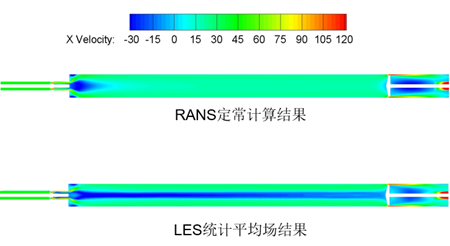(a). Comparison of the overall results of the average velocity field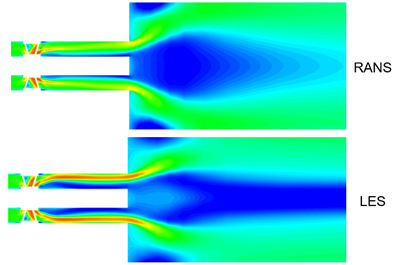(b). Comparison of local results of flow velocity average field

Figure 6  Comparison of average field results of flow velocity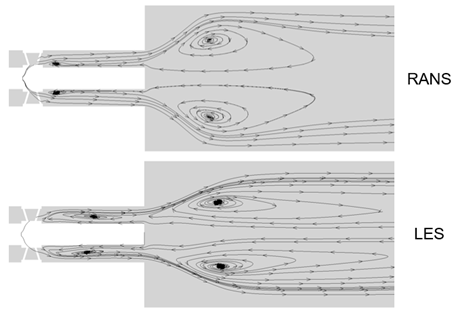Figure 7 Comparison of average speed streamline results

Figure 8 is a comparison of the results of the average temperature field. As can be seen from Figure 8(a), the difference between the average results of RANS and LES mainly exists behind the cyclone and at the entrance of the combustion chamber. As can be seen from Fig. 8(b), in the statistical average result of LES, there will be a significant high temperature region behind the cyclone in the radial inside, but this high temperature is not observed in the calculation result of RANS The existence of the district.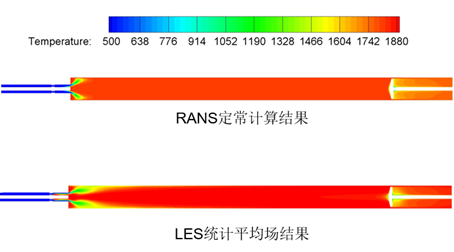(a).  Comparison of the overall results of the average temperature field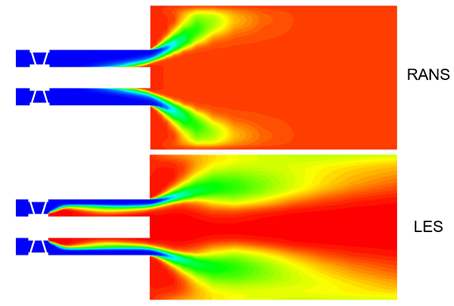(b).  Comparison of local results of average temperature field

Figure 8 Comparison of temperature average field results